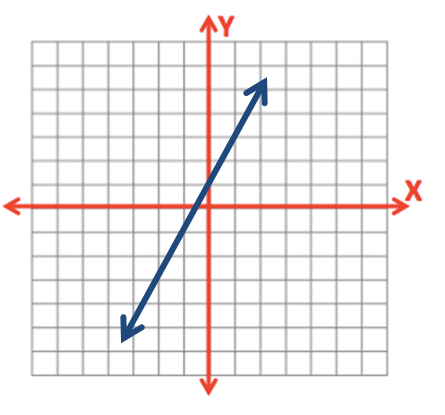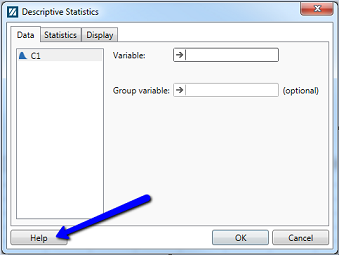# Lesson 0: Prerequisite Skills

Lesson 0: Prerequisite Skills

## What is Statistics?

Statistics
The art and science of answering questions and exploring ideas through the processes of gathering data, describing data, and making generalizations about a population on the basis of a smaller sample.
Statistical literacy

“People’s ability to interpret and critically evaluate statistical information and data-based arguments appearing in diverse media channels, and their ability to discuss their opinions regarding such statistical information” (Gal, as cited by Rumsey, 2002)

Rumsey, D. J. (2002). Statistical literacy as a goal for introductory statistics courses. Journal of Statistics Education, 10(3). Retrieved from: http://www.amstat.org/publications/jse/v10n3/rumsey2.html

Statistical literacy is important because you are faced with statistics problems in your personal and professional lives.

## Examples

Choosing a Medication
Your doctor gives you the option to choose one of two different medications. She provides you with research studies comparing the two medications. How can you use those research studies to inform your decision?

Fantasy Football
In planning for your fantasy football team you come across many tables of statistics. How can you synthesize all of those numbers to best inform your fantasy football draft?

School Curriculum
Your child's school is selecting a new science curriculum. The administration has narrowed it down to three different curricula and is asking parents to vote. What information would you ask for to inform your vote?

Driving to Work
There are two routes that you could take to get to work in the morning. If you go through town, it usually takes between 6 and 14 minutes, depending on the traffic and red lights. If you take the interstate, it consistently takes 10 minutes. Which route will you take to work this morning?

Marketing Decisions
Your company has put you in charge of making a decision between two marketing campaigns. How can you design a research study to collect data to inform your decision?

This is not a mathematics course. While you will need to be able to apply some elementary mathematical procedures (e.g., multiplication, division, exponents, summations), the emphasis of this course is on improving your statistical literacy. On the next page you will review the mathematical operations that we will use this semester.

# 0.1 - Review of Algebra

0.1 - Review of Algebra

Knowledge of the following mathematical operations is required for STAT 200:

• Subtraction
• Division
• Multiplication
• Exponents
• Summations  $\left( \sum \right)$
• Basic linear equations (i.e., $y=mx+b$)

The ability to perform these operations in the appropriate order is necessary. Follow the links below to work through a quick review of order of operations, summations, factorials, and combinations.

We want our students to be successful! In the past, students without knowledge of these topics have struggled to be successful in STAT 200. If you have any questions about these mathematical operations after reviewing these materials, please contact your instructor as soon as possible!

# 0.1.1 - Order of Operations

0.1.1 - Order of Operations

When performing a series of mathematical operations, begin with those inside parentheses or brackets. Next, calculate any exponents or square roots. This is followed by multiplication and division, and finally, addition and subtraction.## Example: Standard Error for Two Proportions

This is a formula that you will see in Lesson 9: $\sqrt{\frac{p_1(1-p_1)}{n_1}+\frac{p_2(1-p_2)}{n_2}}$

$p$ is a proportion and $n$ is a sample size. Let's look at an example of working through this formula with the following values:

$p_1=0.60$

$p_2=0.35$

$n_1=40$

$n_2=80$

We can begin by plugging these values into the formula:

$\sqrt{\frac{0.60(1-0.60)}{40}+\frac{0.35(1-0.35)}{80}}$

The first operations that we perform are the ones in the parentheses:

$\sqrt{\frac{0.60(0.40)}{40}+\frac{0.35(0.65)}{80}}$

Though not typically shown, the values under the square root symbol in the fractions are treated as if they are in parentheses:

$\sqrt{\left ( \frac{0.60(0.40)}{40}\right )+ \left ( \frac{0.35(0.65)}{80}\right ) }$

Working within each set of parentheses, are next step is to multiply in the numerators:

$\sqrt{\left ( \frac{0.24}{40} \right ) + \left ( \frac{0.2275}{80}\right ) }$

Then, the division (i.e., the fractions):

$\sqrt{0.006+0.00284375}$

The addition underneath the square root:

$\sqrt{0.00884375}$

And finally, we take the square root:

$0.0940$

# 0.1.2 - Summations

0.1.2 - Summations

$\Sigma$

This is the Greek capital letter "sigma." In math, this symbol is also known as a summation. This tells us that we should add a series of numbers (i.e., take the sum).

## Example: CandyFour children are comparing how many pieces of candy they have:

ID Child Pieces of Candy
1 Marty 9
2 Harold 8
3 Eugenia 10
4 Kevi 8

We could say that: $x_{1}=9$,$x_{2}=8$, $x_{3}=10$, and $x_{4}=8$.

If we wanted to know how many total pieces of candy the group of children had, we could add the four numbers. The notation for this is:

$\sum x_{i}$

So, for this example, $\sum x_{i}=9+8+10+8=35$

To conclude, combined, the four children have $35$ pieces of candy.

You will first see a summation in Lesson 2 when you learn to compute a sample mean ($\overline{x}$). This is also known as the average. You will learn that $\overline{x}=\frac{\Sigma{X}}{n}$; in other words, the sum of all of the observations divided by the number of observations.

In this example, $\overline{x}=\frac{\Sigma{X}}{n}=\frac{9+8+10+8}{4}=\frac{35}{4}=8.75$

In this sample of four children, the average number of pieces of candy is $8.75$

# 0.1.3 - Basic Linear Equations

0.1.3 - Basic Linear Equations

You may recall from an algebra class that the formula for a straight line is $y=mx+b$ where $m$ is the slope and $b$ is the y-intercept. The x-axis is the horizontal axis and the y-axis is the vertical axis.The y-intercept is where the line crosses the y-axis.  The slope is a measure of change in y over change in x, sometimes written as $\frac{\Delta y}{\Delta x}$ where $\Delta$ ("delta") means change.

## Example

$y=2x+1$

Here, the y-intercept is 1 and the slope is 2.  This line will cross the y-axis at the point (0, 1). From there, for every one increase in $x$, $y$ will increase by 2. In other words, for every one unit we move to the right, we will move up 2.In statistics, the y-intercept is typically written before the slope. The equation for the line above would be written as $y=1+2x$.

Different notation may also be used:

$\widehat y = a + bx$, where $\widehat y$ is the predicted value of y, $a$ is the y-intercept and $b$ is the slope.

$\widehat y = b_0 + b_1 x$, where $\widehat y$ is the predicted value of y, $b_0$ is the y-intercept, and $b_1$ is the slope.

# 0.2 - Introduction to Minitab Express

0.2 - Introduction to Minitab Express

Starting in Lesson 1 we will be using Minitab Express on a regular basis. Take time this week to make sure that you have access to Minitab Express. Minitab Version 17 or 18 is the more complex version of the statistical software however it does NOT provide resampling techniques. Minitab 17 or 18 is NOT sufficient for this course. Minitab Express is required.

#### Minitab Express™

Minitab Express is a statistical software application that was written specifically for introductory STAT courses. It is required software for STAT 200 online. It would not be appropriate for use with graduate level statistics courses.

#### A Quick Introduction to Minitab Express Statistical Software

This introduction to Minitab Express is intended to provide you with enough information to get you started using the basic functionality of Minitab Express. Of course, you will learn more about Minitab Express and its capabilities as you proceed through the course you are taking.

#### Obtaining a Copy of Minitab Express

The Minitab software is available through a number of vendors as well as at minitab.com. You can get a license for 6 or 12 months. Minitab Express is available for both the Mac and PC computing environments. Check the Department of Statistics Statistical Software web page for the latest information.

#### Getting Started with Minitab Express

Here are a couple of videos from Minitab that will familiarize you with the basic components of the application and how they are used in analysis or homework situation.

## Use the 'Help' icons!

As you are using Minitab Express a very helpful feature are the different 'just-in time' access points to Help.  For instance, if you have opened a dialog box for a procedure and are not sure which options to choose, select the 'Help' icon in the lower left.Or, if there is output in the Output window that you don't understand, 'right-click' on the output and select the 'Help' option.Both of these options will open up windows with information that will help you understand the step that you are involved in.

#### Support for Minitab Express

http://support.minitab.com/minitab-express

# 0.3 - Word's Equation Editor

0.3 - Word's Equation Editor

For many of your lab assignments you will be asked to make calculations showing all work. When showing your work you should use the equation editor in Word. The equation editor will look slightly different depending on the version of Word that you are using. The video below walks through a few examples using Microsoft® Word 2016 and Microsoft® Word for Mac 2011.

Word 2016 Equation Editor
Word 2011 Equation Editor

# 0.4 - Canvas' Equation Editor

0.4 - Canvas' Equation Editor

On Canvas discussion boards and essay quiz questions you have the option to "Insert Math Equation"When you click on this button you have the option of switching between "Basic" and "Advanced" views. Most of what you will need to do in STAT 200 can be accomplished under the basic view, with the exception of $\widehat{p}$. The video below will walk you through a few examples.

## Canvas Equation Editor

The table below contains some of the symbols and functions that you will use most often in this course. Note that \widehat will only work in the advanced view.

 Symbol Code $\overline x$ \overline x $\widehat p$ \widehat p $\sum$ \sum $\mu$ \mu $H_0:$ H_0 \$: $s^2$ s^2

  Link ↥ Has Tooltip/Popover Toggleable Visibility